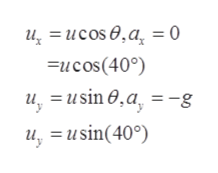Suppose a soccer player boots the ball from a distance 27.5 m into the goal. Find the initial speed of the ball if it just passes over the goal, 2.4 m above the ground, given the intitial direction to be 40° above the horizontal.

Question

Suppose a soccer player boots the ball from a distance 27.5 m into the goal.

Find the initial speed of the ball if it just passes over the goal, 2.4 m above the ground, given the intitial direction to be 40° above the horizontal.

Step 1

Here the figure shows a ball projected from the origin with initial velocity u at an angle θ with the horizontalHere the figure shows a ball projected from the origin with initial velocity u at an angle θ with the horizontal

Step 2

Which has horizontal and vertical components as ux and uy as shown in the diagramhelp_outlineImage Transcriptioncloseu,= ucos0,a = 0 Fucos(40) = usin 0 ,a, -g u, u, usin(40) fullscreen
Step 3

Given,

x=27.5m,

y=2.4m

also, g=9.8m/s2

By using the concept of constant accelerated mot...

Want to see the full answer?

See Solution

Want to see this answer and more?

Our solutions are written by experts, many with advanced degrees, and available 24/7

See Solution
Tagged in

Kinematics